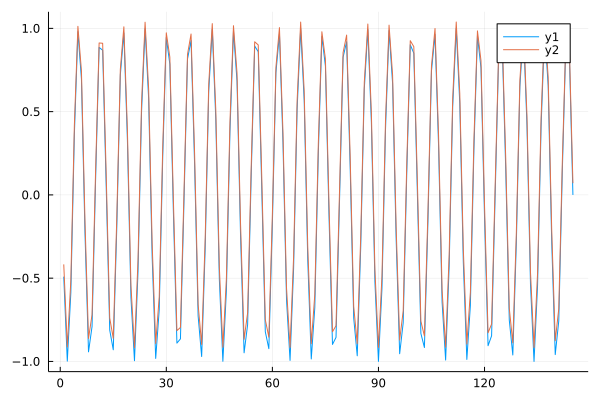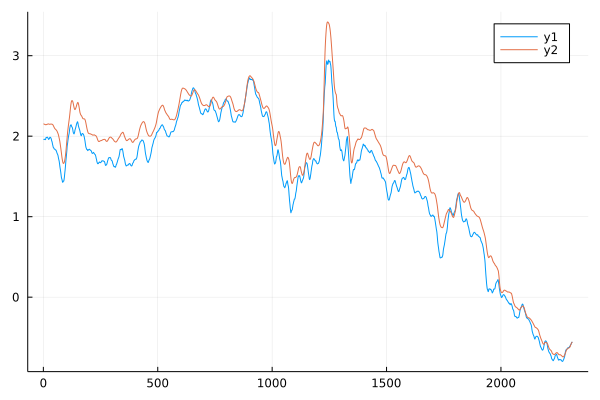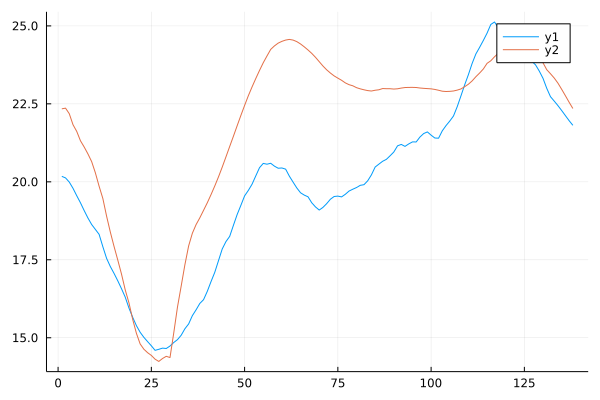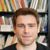## Julia Community 🟣

Matthew leung

Posted on • Updated on

# Use transformer to predict time series

Just tried to use the transformer package to predict time series, details in https://iwasnothing.medium.com/use-transformer-in-julia-8409d2c0b642

Here is the summary:

//
The model can be coded easily using the 2 Julia packages: Flux, and Transformers.jl.

The full code can be found in my Github:
https://github.com/iwasnothing/julia_transformer_ts/blob/main/timeseries-transformer.jl

## Data Preparation

Each training data sample is a sub-sequence of the time series by shifting 1 unit to the right.

## Prediction Testing

For model prediction with the sub-sequence ix, the encoder input (ix[:,1:enc_seq_len,:]), and decoder input by shifting the target by 1 left (ix[:,enc_seq_len:sz-1,:]) were fed into the encoder_forward and decoder_forward to predict the last value (dec[end,:]) in the sequence.

## Test 1 with sin curve

First, I tried to verify the model with simple sin curve data, which it should be predicted well.Both the predicted value and actual value are well fit each other. The result is good.

## Test 2 with interest rate data

I would like to use the actual data to test the model. The data I use is the 10-year daily treasury real yield downloaded since 2003 from the US Treasury. I use 15-day moving average to smoothen the data.Both the predicted value (blue) and actual value (red) are well fit each other. The result is good.

## Test 3 with stock price data

Trying to predict the stock price which is more volatile than interest rate. I use the Julia Package MarketData to get the daily closing stock price. Again, I smoothen the data by taking 15-day moving average.Both the predicted value (blue) and actual value (red) are not well fit each other. The result is not good.